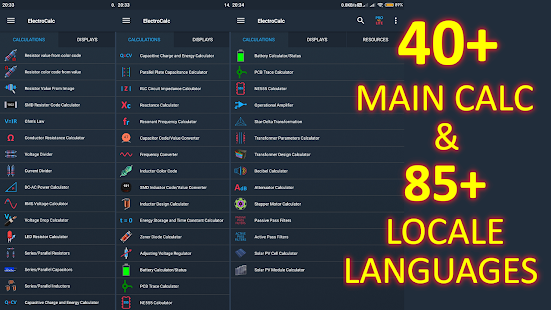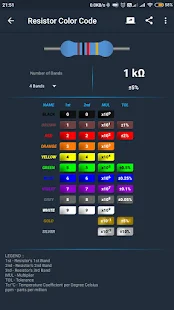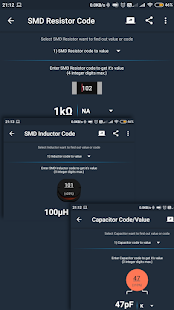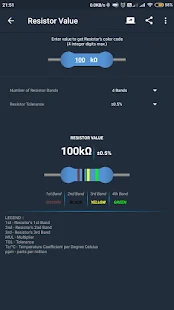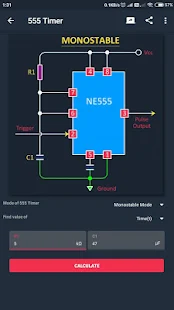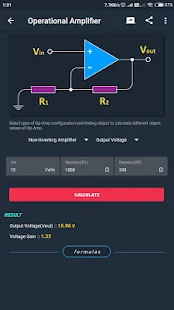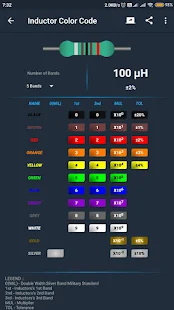Descriptions :

The ElectroCalc application is primarily focused on power electronic circuit calculations for all electronics users. This application is useful for those who are showing interest in electronic circuits that can calculate circuits as below. Each calculation has a circuit image for easy understanding, as well as formulas (in the premium version) of the circuits that can be useful for your DIY jobs.

Features:
- Resistance value of the color code;
- Value resistance color code;
- Image resistance value;
- SMD resistor codes calculator;
- Ohm's law;
- Driver resistance calculator;
- Voltage divider;
- Current divider;
- RMS voltage calculator;
- Voltage drop calculator;
- LED resistor calculator;
- Resistors in series and in parallel;
- Capacitors in series and in parallel;
- Inductors in series and in parallel;
- Capacitive charge and energy calculator;
- Parallel plate capacitance calculator;

Required Android Versions : KitKat [4.4–4.4.4] - Lollipop [5.0–5.0.2] - Marshmallow [6.0 - 6.0.1] - Nougat [7.0 – 7.1.1] - Oreo [8.0-8.1]